# Prologue

## Notation

\begin{align*} V(I) && \text{The variety associated to an ideal } I {~\trianglelefteq~}k[x_1, \cdots, x_{n}] .\end{align*}

# Friday, August 21

General idea: functions in a coordinate ring $$R[x_1, \cdots, x_n]/I$$ will correspond to the geometry of the variety cut out by $$I$$.

• $$x^2 + y^2 - 1$$ defines a circle, say, over $${\mathbb{R}}$$

• $$y^2 = x^3-x$$ gives an elliptic curve: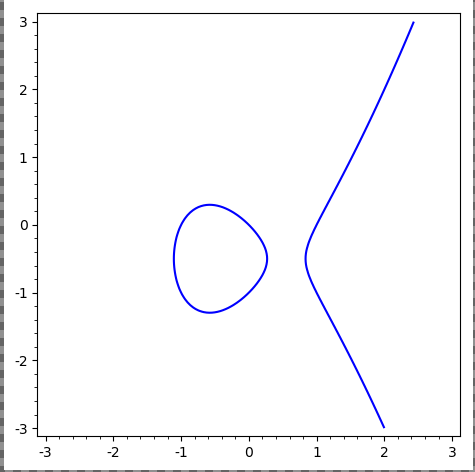• $$x^n+y^n-1$$: does it even contain a $${\mathbb{Q}}{\hbox{-}}$$point? (Fermat’s Last Theorem)

• $$x^2 + 1$$, which has no $${\mathbb{R}}{\hbox{-}}$$points.

• $$x^2 + y^2 + 1/{\mathbb{R}}$$ vanishes nowhere, so its ring of functions is not $${\mathbb{R}}[x, y] / \left\langle{x^2 + y^2 + 1}\right\rangle$$. The problem: $${\mathbb{R}}$$ is not algebraically closed.

• $$x^2 - y^2 = 0$$ over $${\mathbb{C}}$$ is not a manifold (no chart at the origin):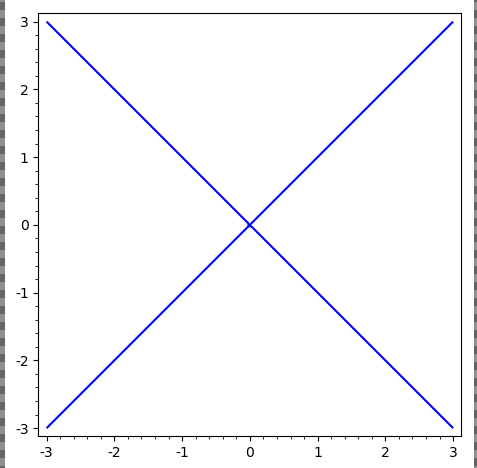• $$x+y+1/{\mathbb{F}}_3$$, which has 3 points over $${\mathbb{F}}_3^2$$, but $$f(x, y) = (x^3 - x)(y^3-y)$$ vanishes at every point

• Not possible when algebraically closed (is there nonzero polynomial that vanishes on every point in $${\mathbb{C}}$$?)

• $$V(f) = {\mathbb{F}}_3^2$$, so the coordinate ring is zero instead of $${\mathbb{F}}_3[x, y]/\left\langle{f}\right\rangle$$ (addressed by scheme theory)

If $$f \in {\mathbb{R}}[x, y]$$ is of degree $$d$$, then[^actual_statement] \begin{align*} \pi_1 V(f) \subseteq {\mathbb{R}}^2 \leq 1 + {(d-1)(d-2) \over 2} \end{align*} [^actual_statement]: Actual statement: the number of connected components is bounded above by this quantity.

Take the curve \begin{align*} X = \left\{{(x, y, z) = (t^3, t^4, t^5) \in {\mathbb{C}}^3 {~\mathrel{\Big|}~}t\in {\mathbb{C}}}\right\} .\end{align*}

Then $$X$$ is cut out by three equations:

• $$y^2 = xz$$
• $$x^2 = yz$$
• $$z^2 = x^2 y$$

Show that the vanishing locus of the first two equations above is $$X\cup L$$ for $$L$$ a line.

Compare to linear algebra: codimension $$d$$ iff cut out by exactly $$d$$ equations.

Given the Riemann surface \begin{align*} y^2 = (x-1)(x-2)\cdots(x-2n) ,\end{align*} how does one visualize its solution set?

On $${\mathbb{C}}$$ with some slits, you can consistently choose a square root of the RHS.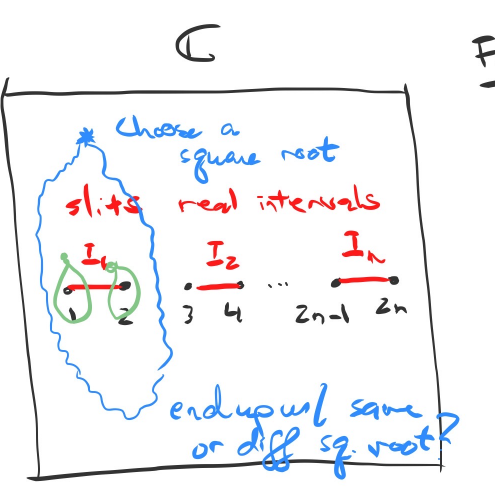Away from $$x=1, \cdots, 2n$$, there are two solutions for $$y$$ given $$x$$.

After gluing along strips, obtain: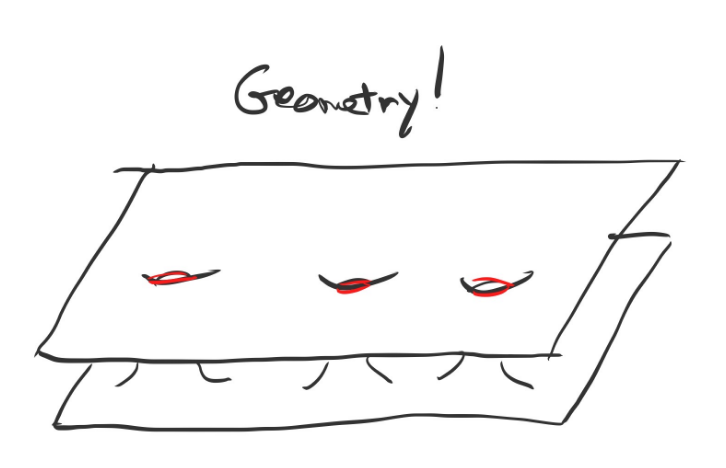# Tuesday, August 25

## Radicals, Degrees, and Affine Varieties

Let $$k = \mkern 1.5mu\overline{\mkern-1.5muk\mkern-1.5mu}\mkern 1.5mu$$ and $$R$$ a ring containing ideals $$I, J$$. Recall the definition of the radical:

The radical of an ideal $$I {~\trianglelefteq~}R$$ is defined as \begin{align*} \sqrt{I} = \left\{{r\in R ~{\text{s.t.}}~r^k\in I \text{ for some } k\in {\mathbb{N}}}\right\} .\end{align*}

Let \begin{align*} I &= (x_1, x_2^2) \subset {\mathbb{C}}[x_1, x_2] \\ &= \left\{{ f_1 x_1 + f_2 x_2 ~{\text{s.t.}}~f_1, f_2 \in {\mathbb{C}}[x_1, x_2]}\right\} \end{align*}

Then $$\sqrt{I} = (x_1, x_2)$$, since $$x_2^2 \in I \implies x_2 \in \sqrt{I}$$.

Given $$f\in k[x_1, \cdots, x_n]$$, take its value at $$a = (a_1, \cdots, a_n)$$ and denote it $$f(a)$$.

Set $$\deg(f)$$ to be the largest value of $$i_1 + \cdots + i_n$$ such that the coefficient of $$\prod x_j ^{i_j}$$ is nonzero.

$$\deg(x_1 + x_2^2 + x_1 x_2^3) = 4$$

1. Affine $$n{\hbox{-}}$$space $${\mathbb{A}}^n = {\mathbb{A}}_k^n$$ is defined as $$\left\{{(a_1, \cdots, a_n) {~\mathrel{\Big|}~}a_i \in k}\right\}$$.1

2. Let $$S\subset k[x_1, \cdots, x_n]$$ to be a set of polynomials. Then define the affine variety of $$S$$ as \begin{align*} V(S) \mathrel{\vcenter{:}}=\left\{{x\in {\mathbb{A}}^n ~{\text{s.t.}}~f(x) = 0}\right\} \subset {\mathbb{A}}^n \end{align*}

• $${\mathbb{A}}^n = V(0)$$.
• For any point $$(a_1, \cdots, a_n)\in {\mathbb{A}}^n$$, then $$V(x_1 - a_1, \cdots, x_n - a_n) = \left\{{a_1, \cdots, a_n}\right\}$$ uniquely determines the point.
• For any finite set $$r_1, \cdots, r_k \in {\mathbb{A}}^1$$, there exists a polynomial $$f(x)$$ whose roots are $$r_i$$.

We may as well assume $$S$$ is an ideal by taking the ideal it generates, \begin{align*} S\subseteq \left\langle{S}\right\rangle = \left\{{\sum g_i f_i {~\mathrel{\Big|}~}g_i \in k[x_1, \cdots, x_n],\, f_i\in S}\right\} .\end{align*} Then $$V(\left\langle{S}\right\rangle) \subset V(S)$$.

Conversely, if $$f_1, f_2$$ vanish at $$x\in {\mathbb{A}}^n$$, then $$f_1 + f_2, gf_1$$ also vanish at $$x$$ for all $$g\in k[x_1, \cdots, x_n]$$. Thus $$V(S) \subset V(\left\langle{S}\right\rangle)$$.

## Ideals, and Properties of $$V({\,\cdot\,})$$

\begin{align*} I+J &\mathrel{\vcenter{:}}=\left\{{f+g ~{\text{s.t.}}~f\in I,\, g\in J}\right\} \\ IJ &\mathrel{\vcenter{:}}=\left\{{\sum_{i=1}^N f_i g_i ~{\text{s.t.}}~f_i\in I,\, g_i\in J, N\in {\mathbb{N}}}\right\} \\ I+J = \left\langle{1}\right\rangle &\implies I\cap J = IJ && \text{(coprime or comaximal)} .\end{align*}

If $$I = \left\langle{a}\right\rangle$$ and $$J = \left\langle{b}\right\rangle$$, then $$I + J = \left\langle{a}\right\rangle + \left\langle{b}\right\rangle = \left\langle{a, b}\right\rangle$$.

1. If $$S_1 \subseteq S_2$$ then $$V(S_1) \supseteq V(S_2)$$.
2. $$V(S_1) \cup V(S_2) = V(S_1 S_2) = V(S_1 \cap S_2)$$.
3. $$\bigcap V(S_i) = V\qty{\bigcup S_i}$$.

We thus have a map \begin{align*} V: \left\{{\text{Ideals in } k[x_1, \cdots, x_n]}\right\} \to \left\{{\text{Affine varieties in } {\mathbb{A}}^n}\right\} .\end{align*}

Let $$X\subset {\mathbb{A}}^n$$ be any set, then the ideal of $$X$$ is defined as \begin{align*} I(X) \mathrel{\vcenter{:}}=\left\{{f\in k[x_1, \cdots, x_n] ~{\text{s.t.}}~f(x) = 0\, \forall x\in X}\right\} .\end{align*}

Let $$X$$ be the union of the $$x_1$$ and $$x_2$$ axes in $${\mathbb{A}}^2$$, then \begin{align*} I(X) = (x_1 x_2) = \left\{{x_1 x_2 g~{\text{s.t.}}~g\in k[x_1, x_2]}\right\} .\end{align*}

If $$X_1 \subset X_2$$ then $$I(X_1) \subset I(X_2)$$.

$$I(X)$$ is a radical ideal, i.e. $$I(X) = \sqrt{I(X)}$$.

This is because $$f(x)^k = 0 \forall x\in X$$ implies $$f(x) = 0$$ for all $$x\in X$$, so $$f^k \in I(X)$$ and thus $$f\in I(X)$$.

Our correspondence is thus \begin{align*} \left\{{\substack{\text{Ideals in } k[x_1, \cdots, x_n]}}\right\} &\xrightarrow{V} \left\{{\substack{\text{Affine Varieties}}}\right\} \\ \left\{{\substack{\text{Radical Ideals}}}\right\} &\xleftarrow{I} \left\{{\substack{\text{?}}}\right\} .\end{align*}

## Statement and Proof of Nullstellensatz

1. For any affine variety $$X$$, \begin{align*} V(I(X)) = X .\end{align*}

2. For any ideal $$J \subset k[x_1, \cdots, x_n]$$, \begin{align*} I(V(J)) = \sqrt{J} .\end{align*}

Thus there is a bijection between radical ideals and affine varieties.

Recall the Hilbert Basis Theorem: any ideal in a finitely generated polynomial ring over a field is again finitely generated.

We need to show 4 inclusions, 3 of which are easy.

1. $$X \subset V(I(X))$$:
• If $$x\in X$$ then $$f(x) = 0$$ for all $$f\in I(X)$$.
• So $$x\in V(I(X))$$, since every $$f\in I(X)$$ vanishes at $$x$$.
1. $$\sqrt{J} \subset I(V(J))$$:
• If $$f\in \sqrt{J}$$ then $$f^k \in J$$ for some $$k$$.
• Then $$f^k(x) = 0$$ for all $$x\in V(J)$$.
• So $$f(x) = 0$$ for all $$x\in V(J)$$.
• Thus $$f\in I(V(J))$$.
1. $$V(I(X)) \subset X$$:
• Need to now use that $$X$$ is an affine variety.
• Counterexample: $$X = {\mathbb{Z}}^2 \subset {\mathbb{C}}^2$$, then $$I(X) = 0$$. But $$V(I(X)) = {\mathbb{C}}^2 \not\subset {\mathbb{Z}}^2$$.
• By (b), $$I(V(J)) \supset \sqrt{J} \supset J$$.
• Since $$V({\,\cdot\,})$$ is order-reversing, taking $$V$$ of both sides reverses the containment.
• So $$V(I(V(J))) \subset V(J)$$, i.e. $$V(I(X)) \subset X$$.

Thus the hard direction that remains is

1. $$I(V(J)) \subset \sqrt{J}$$.

We’ll need the following important theorem:

Any finitely-generated field extension $$k_1 \hookrightarrow k_2$$ is a finite extension of a purely transcendental extension, i.e. there exist $$t_1, \cdots, t_\ell$$ such that $$k_2$$ is finite over $$k_1(t_1, \cdots, t_\ell)$$.

Noether normalization is perhaps more important than the Nullstellensatz!

Suppose $$k$$ is algebraically closed and uncountable2 Then the maximal ideals in $$k[x_1, \cdots, x_n]$$ are of the form $$(x_1 - a_1, \cdots, x_n - a_n)$$.

Let $${\mathfrak{m}}$$ be a maximal ideal, then by the Hilbert Basis Theorem, $${\mathfrak{m}}= \left\langle{f_1, \cdots, f_r}\right\rangle$$ is finitely generated. Let $$L = {\mathbb{Q}}[\left\{{c_i}\right\}]$$ where the $$c_i$$ are all of the coefficients of the $$f_i$$ if $$\operatorname{ch}(K) = 0$$, or $${\mathbb{F}}_p[\left\{{c_i}\right\}]$$ if $$\operatorname{ch}(k) = p$$. Then $$L\subset k$$. Define $${\mathfrak{m}}_0 = {\mathfrak{m}}\cap L[x_1, \cdots, x_n]$$. Note that by construction, $$f_i \in {\mathfrak{m}}_0$$ for all $$i$$, and we can write $${\mathfrak{m}}= {\mathfrak{m}}_0 \cdot k[x_1, \cdots, x_n]$$.

$${\mathfrak{m}}_0$$ is a maximal ideal.

If it were the case that \begin{align*} {\mathfrak{m}}_0 \subsetneq {\mathfrak{m}}_0' \subsetneq L[x_1, \cdots, x_n] ,\end{align*} then \begin{align*} {\mathfrak{m}}_0\cdot k[x_1, \cdots, x_n] \subsetneq {\mathfrak{m}}_0'\cdot k[x_1, \cdots, x_n] \subsetneq k[x_1, \cdots, x_n] .\end{align*}

So far, we’ve constructed a smaller polynomial ring and a maximal ideal in it. Thus $$L[x_1, \cdots, x_n]/{\mathfrak{m}}_0$$ is a field that is finitely generated over either $${\mathbb{Q}}$$ or $${\mathbb{F}}_p$$. So $$L[x_1, \cdots, x_n]/{\mathfrak{m}}_0$$ is finite over some $${\mathbb{Q}}(t_1, \cdots, t_n)$$, and since $$k$$ is uncountable, there exists an embedding $${\mathbb{Q}}(t_1, \cdots, t_n) \hookrightarrow k$$.3

This extends to an embedding of $$\phi: L[x_1, \cdots, x_n]/{\mathfrak{m}}_0 \hookrightarrow k$$ since $$k$$ is algebraically closed. Letting $$a_i$$ be the image of $$x_i$$ under $$\phi$$, then $$f(a_1, \cdots, a_n) = 0$$ by construction, $$f_i \in (x_i - a_i)$$ implies that $${\mathfrak{m}}= (x_i - a_i)$$ by maximality.

# Thursday, August 27

## Consequence of the Nullstellensatz

Recall Hilbert’s Nullstellensatz:

1. For any affine variety, $$V(I(X)) = X$$.

2. For any ideal $$J{~\trianglelefteq~}k[x_1, \cdots, x_n]$$, $$I(V(J)) = \sqrt{J}$$.

So there’s an order-reversing bijection \begin{align*} \left\{{\substack{\text{Radical ideals } k[x_1, \cdots, x_n]}}\right\} \to{V({\,\cdot\,})}{I({\,\cdot\,})} \left\{{\substack{\text{Affine varieties in } {\mathbb{A}}^n}}\right\} .\end{align*}

In proving $$I(V(J)) \subseteq \sqrt{J}$$, we had an important lemma (Noether Normalization): the maximal ideals of $$k[x_1, \cdots, x_n]$$ are of the form $$\left\langle{x-a_1, \cdots, x-a_n}\right\rangle$$.

If $$V(I)$$ is empty, then $$I = \left\langle{1}\right\rangle$$.

This is because no common vanishing locus $$\implies$$ trivial ideal, so there’s a linear combination that equals 1.

The only ideals that vanish nowhere are trivial.

By contrapositive, suppose $$I\neq \left\langle{1}\right\rangle$$. By Zorn’s Lemma, these exists a maximal ideals $${\mathfrak{m}}$$ such that $$I \subset {\mathfrak{m}}$$. By the order-reversing property of $$V({\,\cdot\,})$$, $$V({\mathfrak{m}}) \subseteq V(I)$$. By the classification of maximal ideals, $${\mathfrak{m}}= \left\langle{x-a_1, \cdots, x-a_n}\right\rangle$$, so $$V({\mathfrak{m}}) = \left\{{a_1, \cdots, a_n}\right\}$$ is nonempty.

Returning to the proof that $$I(V(J)) \subseteq \sqrt{J}$$: let $$f\in V(I(J))$$, we want to show $$f\in \sqrt{J}$$. Consider the ideal $$\tilde J \mathrel{\vcenter{:}}= J + \left\langle{ft - 1}\right\rangle \subseteq k[x_1, \cdots, x_n, t]$$.

$$f = 0$$ on all of $$V(J)$$ by the definition of $$I(V(J))$$.

However, if $$f=0$$, then $$ft-1 \neq 0$$, so $$V(\tilde J) = V(G) \cap V(ft-1) = \emptyset$$.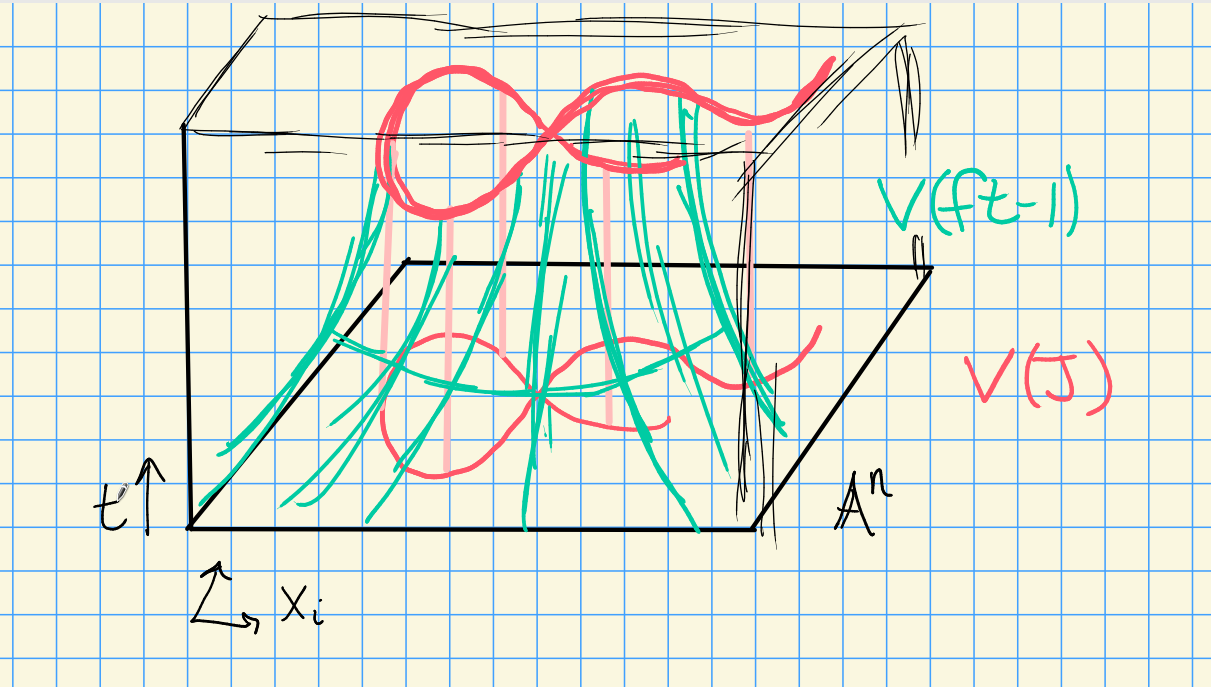Effect, a hyperbolic tube around $$V(J)$$, so both can’t vanish

Applying the corollary $$\tilde J = (1)$$, so \begin{align*} 1 = \left\langle{ft-1}\right\rangle g_0(x_1, \cdots, x_n, t) + \sum f_i g_i(x_1, \cdots, x_n, t) \end{align*} with $$f_i \in J$$. Let $$t^N$$ be the largest power of $$t$$ in any $$g_i$$. Thus for some polynomials $$G_i$$, we have \begin{align*} f^N \mathrel{\vcenter{:}}=(ft-1) G_0(x_1, \cdots, x_n, ft) + \sum f_i G_i(x_1, \cdots, x_n, ft) \end{align*} noting that $$f$$ does not depend on $$t$$. Now take $$k[x_1, \cdots, x_n, t]/\left\langle{ft-1}\right\rangle$$, so $$ft=1$$ in this ring. This kills the first term above, yielding \begin{align*} f^N = \sum f_i G_i(x_1, \cdots, x_n, 1) \in k[x_1, \cdots, x_n, t]/\left\langle{ft-1}\right\rangle .\end{align*}

There is an inclusion \begin{align*} k[x_1, \cdots, x_n] \hookrightarrow k[x_1, \cdots, x_n, t]/\left\langle{ft-1}\right\rangle .\end{align*}

Why is this true?

Since this is injective, this identity also holds in $$k[x_1, \cdots, x_n]$$. But $$f_i\in J$$, so $$f\in \sqrt{I}$$.

Consider $$k[x]$$. If $$J\subset k[x]$$ is an ideal, it is principal, so $$J = \left\langle{f}\right\rangle$$. We can factor $$f(x) = \prod_{i=1}^k (x-a_i)^{n_i}$$ and $$V(f) = \left\{{a_1, \cdots, a_k}\right\}$$. Then \begin{align*} I(V(f)) = \left\langle{(x-a_1)(x-a_2)\cdots(x-a_k)}\right\rangle = \sqrt{J} \subsetneq J ,\end{align*} so this loses information.

Let $$J = \left\langle{x-a_1, \cdots, x-a_n}\right\rangle$$, then $$I(V(J)) = \sqrt{J} = J$$ with $$J$$ maximal. Thus there is a correspondence \begin{align*} \left\{{\substack{\text{Points of } {\mathbb{A}}^n}}\right\} \iff \left\{{\substack{\text{Maximal ideals of }k[x_1, \cdots, x_n]}}\right\} .\end{align*}

1. $$I(X_1 \cup X_2) = I(X_1) \cap I(X_2)$$.

2. $$I(X_1) \cap I(X_2) = \sqrt{I(X_1) + I(X_2)}$$.

We proved (a) on the variety side.

For (b), by the Nullstellensatz, $$X_i = V(I(X_i))$$, so \begin{align*} I(X_1\cap X_2) &= I\qty{ VI(X_1) \cap VI(X_2)} \\ &= IV\qty{I(X_1) + I(X_2)} \\ &= \sqrt{I(X_1) + I(X_2)} .\end{align*}

Example of property (b):

Take $$X_1 = V(y-x^2)$$ and $$X_2 = V(y)$$, a parabola and the $$x{\hbox{-}}$$axis.

Then $$X_1 \cap X_2 = \left\{{(0, 0)}\right\}$$, and $$I(X_1) + I(X_2) = \left\langle{y-x^2, y}\right\rangle = \left\langle{x^2, y}\right\rangle$$, but \begin{align*} I(X_1 \cap X_2) = \left\langle{x, y}\right\rangle = \sqrt{\left\langle{x^2, y}\right\rangle} \end{align*}

If $$f, g\in k[x_1, \cdots, x_n]$$, and suppose $$f(x) = g(x)$$ for all $$x\in {\mathbb{A}}^n$$. Then $$f = g$$.

Since $$f-g$$ vanishes everywhere, $$f-g \in I({\mathbb{A}}^n) = I(V(0)) = \sqrt{0} = 0$$.

More generally suppose $$f(x) = g(x)$$ for all $$x\in X$$, where $$X$$ is some affine variety. Then by definition, $$f-g \in I(X)$$, so a “natural” space of functions on $$X$$ is $$k[x_1,\cdots, x_n]/I(X)$$.

For an affine variety $$X$$, the coordinate ring of $$X$$ is \begin{align*} A(X) \mathrel{\vcenter{:}}= k[x_1, \cdots, x_n]/ I(X) .\end{align*}

Elements $$f\in A(X)$$ are called polynomial or regular functions on $$X$$.

The constructions $$V({\,\cdot\,}), I({\,\cdot\,})$$ work just as well for $$A(X)$$ and $$X$$.

Given any $$S\subset A(Y)$$ for $$Y$$ an affine variety, \begin{align*} V(S) = V_Y(S) \mathrel{\vcenter{:}}=\left\{{x\in Y ~{\text{s.t.}}~f(x) = 0\,\,\forall f\in S}\right\} .\end{align*}

Given $$X\subset Y$$ a subset, \begin{align*} I(X) = I_Y(X) \mathrel{\vcenter{:}}=\left\{{f\in A(Y) ~{\text{s.t.}}~f(x) = 0\,\,\forall x\in X}\right\} \subseteq A(Y) .\end{align*}

For $$X\subset Y \subset {\mathbb{A}}^n$$, we have $$I(X) \supset I(Y) \supset I({\mathbb{A}}^n)$$, so we have mapsLet $$X\subset Y$$ be an affine subvariety, then

1. $$A(X) = A(Y) / I_Y(X)$$

2. There is a correspondence \begin{align*} \left\{{\substack{\text{Affine subvarieties of }Y}}\right\} &\iff \left\{{\substack{\text{Radical ideals in }A(Y)}}\right\} \\ X &\mapsto I_Y(X) \\ V_Y(J) &\mapsfrom J .\end{align*}

Properties are inherited from the case of $${\mathbb{A}}^n$$, see exercise in Gathmann.

Let $$Y = V(y-x^2) \subset {\mathbb{A}}^2/{\mathbb{C}}$$ and $$X = \left\{{(1, 1)}\right\} = V(x-1, y-1)\subset {\mathbb{A}}^2/{\mathbb{C}}$$.

Then there is an inclusion $$\left\langle{y-x^2}\right\rangle \subset \left\langle{x-1, y-1}\right\rangle$$ (e.g. by Taylor expanding about the point $$(1, 1)$$), and there is a map# Tuesday, September 01

Last time: $$V(I) = \left\{{x\in {\mathbb{A}}^n ~{\text{s.t.}}~f(x) = 0 \, \forall x\in I}\right\}$$ and $$I(X) = \left\{{f\in k[x_1, \cdots, x_n] ~{\text{s.t.}}~f(x) = 0\, \forall x\in X}\right\}$$. We proved the Hilbert Nullstellensatz $$I(V(J)) = \sqrt{J}$$, defined the coordinate ring of an affine variety $$X$$ as $$A(X) \mathrel{\vcenter{:}}= k[x_1, \cdots, x_n] / I(X)$$, the ring of “regular” (polynomial) functions on $$X$$.

Recall that a topology on $$X$$ can be defined as a collection of “closed” subsets of $$X$$ that are closed under arbitrary intersections and finite unions. A subset $$Y\subset X$$ inherits a subspace topology with closed sets of the form $$Z\cap Y$$ for $$Z\subset X$$ closed.

Let $$X$$ be an affine variety. The closed sets are affine subvarieties $$Y\subset X$$.

We have $$\emptyset, X$$ closed, since

1. $$V_X(1) = \emptyset$$,
2. $$V_X(0) = X$$

Closure under finite unions: Let $$V_X(I), V_X(J)$$ be closed in $$X$$ with $$I, J \subset A(X)$$ ideals. Then $$V_X(IJ) = V_X(I) \cup V_X(J)$$.

Closure under intersections: We have $$\bigcap_{i\in \sigma} V_X(J) = V_X\qty{ \sum_{i\in \sigma} J_i}$$.

There are few closed sets, so this is a “weak” topology.

Compare the classical topology on $${\mathbb{A}}^1/{\mathbb{C}}$$ to the Zariski topology.

Consider the set $$A\mathrel{\vcenter{:}}=\left\{{x\in {\mathbb{A}}^1/{\mathbb{C}}~{\text{s.t.}}~{\left\lVert {x} \right\rVert} \leq 1}\right\}$$, which is closed in the classical topology.

But $$A$$ is not closed in the Zariski topology, since the closed subsets are finite sets or the whole space.

Here the topology is in fact the cofinite topology.

Let $$f: {\mathbb{A}}^1/k\to {\mathbb{A}}^1/k$$ be any injective map. Then $$f$$ is necessarily continuous wrt the Zariski topology.

Thus the notion of continuity is too weak in this situation.

Consider $$X\times Y$$ a product of affine varieties. Then there is a product topology where open sets are of the form $$\bigcup_{i=1}^n U_i \times V_i$$ with $$U_i, V_i$$ open in $$X, Y$$ respectively.

This is the wrong topology! On $${\mathbb{A}}^1 \times{\mathbb{A}}^1 = {\mathbb{A}}^2$$, the diagonal $$\Delta \mathrel{\vcenter{:}}= V(x-y)$$ is closed in the Zariski topology on $${\mathbb{A}}^2$$ but not in the product topology.

Consider $${\mathbb{A}}^2/{\mathbb{C}}$$, so the closed sets are curves and points. Observation: $$V(x_1 x_2 ) \subset {\mathbb{A}}^2/{\mathbb{C}}$$ decomposed into the union of the coordinate axes $$X_1 \mathrel{\vcenter{:}}= V(x_1)$$ and $$X_2 \mathrel{\vcenter{:}}= V(x_2)$$. The Zariski topology can detect these decompositions.

Let $$X$$ be a topological space.

1. $$X$$ is reducible iff there exist nonempty proper closed subsets $$X_1 ,X_2 \subset X$$ such that $$X = X_1 \cup X_2$$. Otherwise, $$X$$ is said to be irreducible.

2. $$X$$ is disconnected if there exist $$X_1, X_2 \subset X$$ such that $$X = X_1 {\coprod}X_2$$. Otherwise, $$X$$ is said to be connected.

$$V(x_1 x_2)$$ is reducible but connected.

$${\mathbb{A}}^1/{\mathbb{C}}$$ is not irreducible, since we can write $${\mathbb{A}}^1/{\mathbb{C}}= \left\{{{\left\lVert {x} \right\rVert} \leq 1}\right\} \cup\left\{{{\left\lVert {x} \right\rVert} \geq 1}\right\}$$.

Let $$X$$ be a disconnected affine variety with $$X = X_1 {\coprod}X_2$$. Then $$A(X) \cong A(X_1) \times A(X_2)$$.

We have $$X_1 \cup X_2 = X$$, so $$I(X_1) \cap I(X_2) = I(X) = (0)$$ in the coordinate ring $$A(X)$$ (recalling that it is a quotient by $$I(X)$$.)

Since $$X_1 \cap X_1 \emptyset$$, we have \begin{align*} I(X_1 \cap X_2) = \sqrt{I(X_1) + I(X_2) } = I(\emptyset) = \left\langle{1}\right\rangle .\end{align*}

Thus $$I(X_1) + I(X_2) = \left\langle{1}\right\rangle$$, and by the Chinese Remainder Theorem, the following map is an isomorphism: \begin{align*} A(X) \to A(X)/I(X_1) \times A(X) / I(X_2) .\end{align*}

But the codomain is precisely $$A(X_1) \times A(X_2)$$.

An affine variety $$X$$ is irreducible $$\iff$$ $$A(X)$$ is an integral domain.

$$\implies$$: By contrapositive, suppose $$f_1, f_2 \in A(X)$$ are nonzero with $$f_1 f_2 = 0$$. Let $$X_i = V(f_i)$$, then $$X= V(0) = V(f_1 f_2) = X_1 \cup X_2$$ which are closed and proper since $$f_i \neq 0$$.

$$\impliedby$$: Suppose $$X$$ is reducible with $$X = X_1 \cup X_2$$ with $$X_i$$ proper and closed. Define $$J_i \mathrel{\vcenter{:}}= I(X_i)$$, and note $$J_i \neq 0$$ because $$V(J_i) = V(I(X_i)) = X_i$$ by part (a) of the Nullstellensatz.

So there exists a nonzero $$f_i \in J_i = I(X_i)$$, so $$f_i$$ vanishes on $$X_i$$. But then $$V(f_1) \cup V(f_2) \supset X_1 \cup X_2 = X$$, so $$X= V(f_1 f_2)$$ and $$f_1 f_2 \in I(X) = \left\langle{0}\right\rangle$$ and $$f_1 f_2 = 0$$. So $$A(X)$$ is not a domain.

Let $$X = \left\{{p_1, \cdots, p_d}\right\}$$ be a finite set in $${\mathbb{A}}^n$$. The Zariski topology on $$X$$ is the discrete topology, and $$X = {\coprod}\left\{{p_i}\right\}$$. So \begin{align*} A(X) = A({\coprod}\left\{{p_i}\right\}) = \prod_{i=1}^d A({\left\{{p_i}\right\}}) = \prod_{i=1}^d k[x_1, \cdots, x_n] / \left\langle{x_j - a_j(p_i)}\right\rangle_{j=1}^d .\end{align*}

Set $$V(x_1 x_2) = X$$, then $$A(X) = k[x_1, x_2]/ \left\langle{x_1 x_2}\right\rangle$$. This not being a domain (since $$x_1 x_2 = 0$$) corresponds to $$X = V(x_1) \cup V(x_2)$$ not being irreducible.

$${\mathbb{A}}^2/k$$ is irreducible since $$k[x_1, \cdots x_n]$$ is a domain.

Let $$X_1$$ be the $$xy$$ plane and $$X_2$$ be the line parallel to the $$y{\hbox{-}}$$axis through $${\left[ {0,0,1} \right]}$$, and let $$X= X_1 {\coprod}X_2$$. Then $$X_1 = V(z)$$ and $$X_2 = V(x, z-1)$$, and $$I(X) = \left\langle{z}\right\rangle \cdots \left\langle{x, z-1}\right\rangle= \left\langle{xz, z^2 - z}\right\rangle$$.

Then the coordinate ring is given by $$A(X) = {\mathbb{C}}[x, y, z] / \left\langle{xz, z^2 - z}\right\rangle = {\mathbb{C}}[x, y, z] / \left\langle{z}\right\rangle \oplus {\mathbb{C}}[x, y,z] / \left\langle{x, z-1}\right\rangle$$.

# Thursday, September 03

Recall that the Zariski topology is defined on an affine variety $$X = V(J)$$ with $$J {~\trianglelefteq~}k[x_1, \cdots, x_n]$$ by describing the closed sets.

$$X$$ is irreducible if its coordinate ring $$A(X)$$ is a domain.

There is a 1-to-1 correspondence \begin{align*} \left\{{\substack{\text{Irreducible subvarieties} \\ \text{of }X}}\right\} \iff \left\{{\substack{\text{Prime ideals} \\ \text{in }A(X)}}\right\} .\end{align*}

Suppose $$Y\subset X$$ is an affine subvariety. Then \begin{align*} A(X) / I_X(Y) = A(Y) .\end{align*}

By NSS, there is a bijection between subvarieties of $$X$$ and radical ideals of $$A(X)$$ where $$Y\mapsto I_X(Y)$$. A quotient is a domain iff quotienting by a prime ideal, so $$A(Y)$$ is a domain iff $$I_X(Y)$$ is prime.

Recall that $${\mathfrak{p}}{~\trianglelefteq~}R$$ is prime when $$fg\in {\mathfrak{p}}\iff f\in {\mathfrak{p}}$$ or $$g\in {\mathfrak{p}}$$. Thus $$\mkern 1.5mu\overline{\mkern-1.5muf\mkern-1.5mu}\mkern 1.5mu \mkern 1.5mu\overline{\mkern-1.5mug\mkern-1.5mu}\mkern 1.5mu = 0$$ in $$R/{\mathfrak{p}}$$ implies $$\mkern 1.5mu\overline{\mkern-1.5muf\mkern-1.5mu}\mkern 1.5mu = 0$$ or $$\mkern 1.5mu\overline{\mkern-1.5mug\mkern-1.5mu}\mkern 1.5mu = 0$$ in $$R/{\mathfrak{p}}$$, i.e. $$R/{\mathfrak{p}}$$ is a domain.

Finally note that prime ideals are radical (easy proof).

Consider $${\mathbb{A}}^2/{\mathbb{C}}$$ and some subvarieties $$C_i$$: